“It turns the dark and strange arts of shader programming into something understandable and non-evil”
Nicklas 'Nifflas' Nygren, developer of the Knytt series

Search Box

Eng - 简体字 - 正體字
Beginner's tutorial
Main
 This is the node in which all your other nodes in the end link to. It has several inputs that all serve different purposes. The animated images below show all of the inputs and how they behave when changed over time. Most of them animate back and forth between 0 and 1 (Black and White)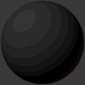DiffuseThis is the main color of your shaders. The diffuse color will receive light, have light falloff depending on the light-normal angle, and be shaded by shadows.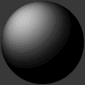Diffuse PowerThis is the exponent of the falloff of the light-normal angle. Can be used to get an extra metallic look when using values above 1. Note that this does not currently conserve energy, whenever that is enabled.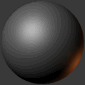SpecularThis is the color of the specular highlights of your shader. Higher values are brighter, black will not affect the shader at all.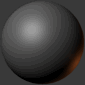GlossThis is the exponent of the specular highlights. Higher values will make it look shiny, values approaching 0 will make it look matte. Note that if you have unchecked gloss remapping, you should avoid using gloss values below 1.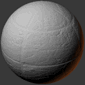NormalThis is the tangent-space normal direction, where you can connect normal maps or custom normal vectors.EmissionThis is simply light that is always added to your shader, regardless of the lighting conditions.TransmissionThis controls how much light is passed through when the light source is behind the surface currently being rendered. This can be useful for thin materials such as cloth or vegetation.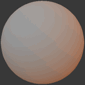Light WrappingThis is a way of controlling the light-normal angle falloff offset, which can be used to get an effect similar to subsurface scattering. Works best for smooth objects. Inputting a red-ish value will make the red channel "wrap around" the object more than the others, making it look as if light passed into the mesh, and came out with a red wavelength, similar to how skin is shaded.Diffuse Ambient LightThis adds light to your shader, affected by your diffuse. Can be used with, for example, cubemap using the normal direction for image-based lighting (IBL), or ambient light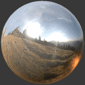Specular Ambient LightThis adds light to your shader, affected by your specular. Can be used with, for example, a cubemap using the view reflection direction for image-based lighting (IBL)Diffuse Ambient OcclusionThis dampens indirect diffuse light, such as light probes, indirect light, and diffuse ambient lightSpecular Ambient OcclusionThis dampens indirect specular light, such as reflection probes, and specular ambient lightCustom LightingThis input is active when your shader is set to unlit, allowing you to define custom lighting behaviour. The nodes you put here are per-light.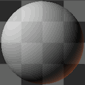OpacityOpacity controls the transparency of the final pixel. Note that partial transparency is generally finicky to get right, especially when using deferred rendering.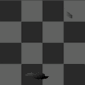Opacity ClipOpacity Clip is a way of controlling if the current pixel/fragment should draw or not. Always use Opacity Clip for objects that need transparency, but not partial transparency, as Opacity Clip is easily sorted, which Opacity is not.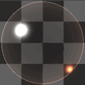RefractionRefraction is a screen-space UV offset for refracting the background pixels. Make sure you set the alpha to something below 1 before using, so that the refraction effect is visible.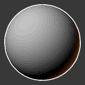Outline WidthThis will add an outline to your shader, rendered as an offset mesh with reversed face normals. Note that hard edges will break the outline.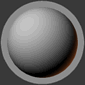Outline ColorThis controls the color of the outline.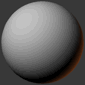Vertex OffsetThis can be used to animate shaders over time, or change the shape of the object in various conditions. You simply insert a XYZ coordinate for how much each vertex should be offset.DX11 DisplacementThis works very much in the same way as Vertex Offset, but is used in conjunction with DX11 tessellation. (Note that DirectX is Windows only, requires a DX11 GPU and has to be enabled in Unity)DX11 TessellationThis controls how many subdivisions you want to split your triangles into. (Note that DirectX is Windows only, requires a DX11 GPU and has to be enabled in Unity)
Add
A + LMB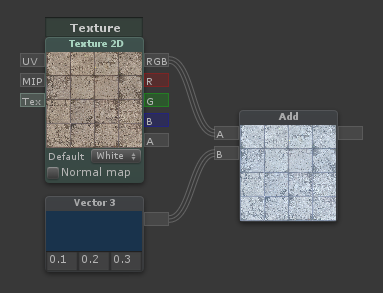Outputs the sum [A] + [B]
Subtract
S + LMBOutputs the difference [A] - [B]
Multiply
M + LMBOutputs the product [A] * [B]
Divide
D + LMBOutputs the quotient [A] / [B]
Reciprocal
 Outputs the quotient 1 / input
Power
E + LMBOutputs the power [Val] ^ [Exp]
Sqrt
 Outputs the square root of its input
Log
 Outputs the logarithm of its input. You can switch log base in the dropdown menu
Min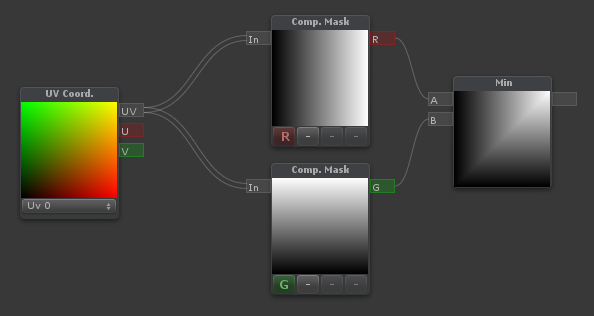Outputs the minimum of [A] and [B]
Max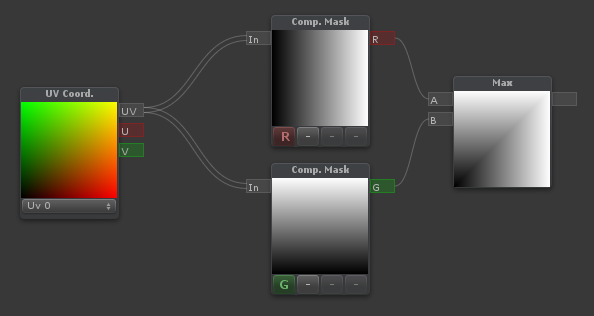Outputs the maximum of [A] and [B]
Abs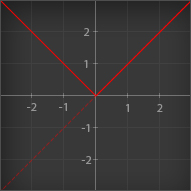Outputs the absolute value of its input. Essentially; it makes negative values positive
SignOutputs the sign of its input. Values greater than 0 outputs 1 Values equal to 0 outputs 0 Values less than 0 outputs -1
Ceil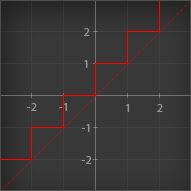Outputs its input rounded up to the nearest integer
Round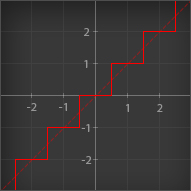Outputs its input rounded to the nearest integer
Floor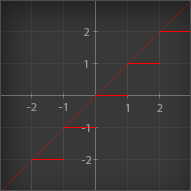Outputs its input rounded down to the nearest integer
Trunc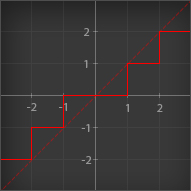Outputs its input rounded to the nearest integer towards zero. Essentially; it removes the decimals, leaving an integer
Step (A <= B)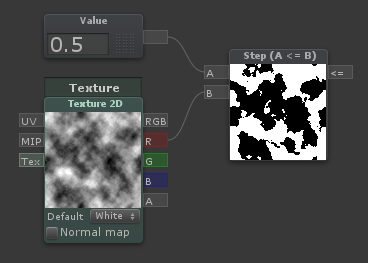Outputs 1 if [A] is less than or equal to [B], otherwise outputs 0
Smoothstep
 Blends smoothly between two values, based on where a third value is in that range, outputting values between 0 and 1. Think of it as a clamped inverse lerp with a smoothed output value.
If
I + LMB
 Outputs the [A>B] input when [A] is greater than [B] Outputs the [A=B] input when [A] is equal to [B] Outputs the [A
FracOutputs the fractional part of its input. Essentially; it removes the integer part and keeps only the decimal part. An input of 4.32 would output 0.32. This node is particularly useful in conjunction with the Time node, which gives you a sawtooth wave over time
Fmod
 Outputs the remainder of [A] divided by [B]
Clamp
 Outputs its main input value, no less than [Min] and no more than [Max]
Clamp (Simple)
 The same as Clamp, but with two numerical inputs for Min and Max instead of node connectors
Clamp 0-1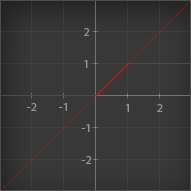Outputs its input value, no less than 0 and no more than 1
Lerp
L + LMB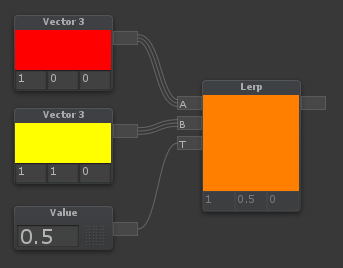Lerp is used to blend between two values or colors. If [T] is 0, it will output [A] If [T] is 0.5, it will output a halfway blend between [A] and [B] If [T] is 1, it will output [B] If [T] is any other value, it will output a linear blend of the two.
Lerp (Simple)
 The same as Lerp, but with two numerical inputs for [A] and [B] instead of node connectors
InverseLerpInverseLerp is usually used to remap ranges. If [T] is equal to [A], it will output 0 If [T] is halfway between [A] and [B], it will output 0.5 If [T] is equal to [B], it will output 1 If [T] is any other value, it will output a linear blend of the two.
Posterize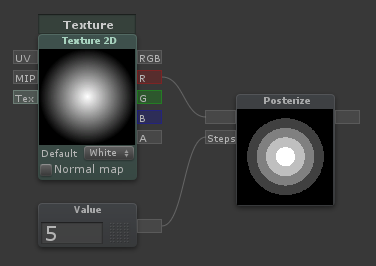Rounds values based on the value coming through [Steps]. A [Steps] value of 5 will create 5 bands in the 0 to 1 range
Blend
B + LMB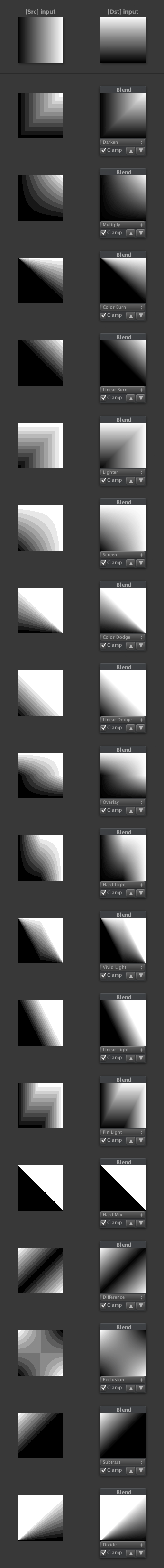Blends A over B using the specified method
RemapRemaps a value from one range to another. Same as Remap (Simple), but with inputs instead of numerical constants
Remap (Simple)
R + LMB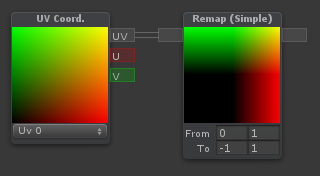Remaps a value from one range to another. For instance, if the node expects values from -1 to 1, but you want it to output a value from 2 to 5, you can type -1 and 1 on the first line, 2 and 5 on the second line
Noise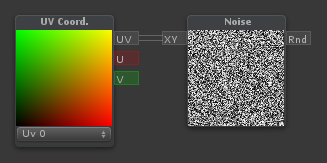Generates pseudorandom numbers based on a two-component input (Such as UV coordinates)
One Minus
O + LMB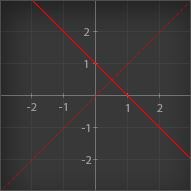Outputs 1 minus its input. When used with color inputs, it will invert the color
Negate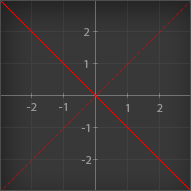Outputs the main input multiplied by -1. Essentially makes positive values negative, and negative values positive
Exp
 When Exp is selected: Outputs e to the power of its input When Exp 2 is selected: Outputs 2 to the power of its input
Hue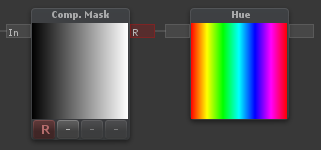Outputs an RGB color given a Hue
HSV to RGB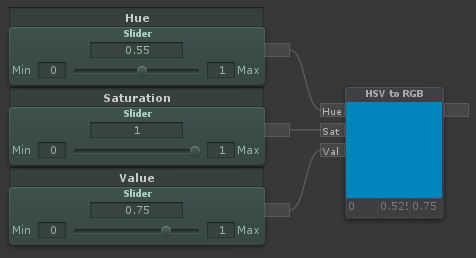Outputs an RGB color given a HSV (Hue, Saturation and Value) input. Hue and Saturation are between 0 and 1. Value is too, but can go beyond 1 to overexpose colors
RGB to HSVOutputs HSV (Hue, Saturation and Value) components given a color input. Hue and Saturation are between 0 and 1. Value can go beyond 1 for overexposed colors
Value
1 + LMB
 A numerical value, can also be called a "Vector 1". A property version is also available. Values can be used with the Append node to create Vectors with more components. Values can also be multiplied with vectors/colors. For example, a vector (3,1,0) multiplied by a value of 0.5, outputs the vector (1.5,0.5,0)
Vector 2
2 + LMB
 A vector with two components/values. Usually used with UV coordinates. Adding a Vector 2 to UV coordinates, will translate the UVs. Multiplying UV coordinates with a Vector 2 will scale the UVs
Vector 3
3 + LMB
 A vector with three components/values. Usually used as a color, position or direction
Vector 4
4 + LMB
 A vector with four components/values. Usually used as a color with an alpha channel, or as a position with some extra data in the fourth channel. There are two parameters to expose in the inspector available. Color and Vector 4 parameter
Texture 2D
T + LMB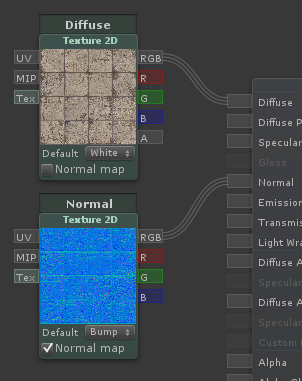Contains a reference to a texture and will sample a texture at a specific UV coordinate with a specific MIP level (if connected). If the [Tex] input is connected by a Texture Asset node, this will no longer be a parameter in the inspector. Outputs [RGB] as well as separate channels
Texture Asset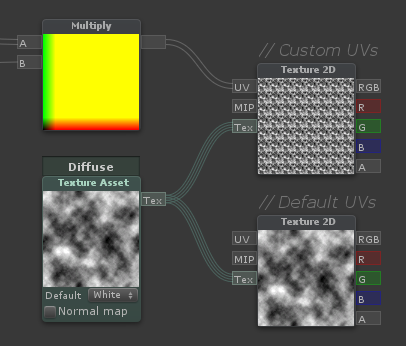Contains a reference to a texture. This is used to sample a single texture multiple times - Can only be connected to the [Tex] input of Texture 2D nodes. This will also be reflected in the inspector of the material, so the user only need to assign one texture
Value (Property)
 A numerical value; same as Value, but exposed in the material inspector
Vector 4 (Property)
 A vector with four components/values, same as Vector 4, but exposed in the material inspector as 4 separate X, Y, Z and W values
Color
 A vector with four components/values, same as Vector 4, but exposed in the material inspector with a color picker
Cubemap
 Contains a reference to a cubemap and will sample a it in a specific direction with a specific MIP level (If connected). Outputs [RGB] as well as separate channels
Slider
 Allows you to easily tweak a value between a min and a max value. Is also exposed to the inspector
Switch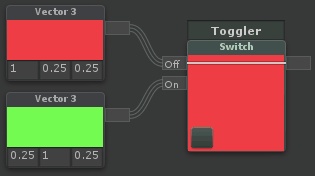Switches between two inputs based on a checkbox. Note that this cannot be used to optimize a shader, it will process both branches, but only show one
Toggle
 A value that is either 0 or 1 based on a checkbox
Dot Product
 Outputs the Dot product between [A] and [B]. Essentially; for two normalized vectors, it outputs how far they point away from each other. If they point in the same direction, it outputs 1, if they are perpendicular to each other, it outputs 0, if they point in opposite directions, it outputs -1. Dropdown selections: Standard - Regular Dot Product Positive - Makes all negative values 0 Negative - Makes all positive values 0 Abs - Makes all negative values positive Normalized - Outputs in the range 0 to 1 instead of -1 to 1 The graph below show how the different modes behave when using two normalized vectors. On the X axis you have the angle between them, on the Y axis you have the output value:Cross Product
 Outputs the Cross product of [A] and [B]. Essentially; it outputs a vector perpendicular to both input vectors
Reflect
 Outputs the reflection vector of an incoming vector [I] as if reflected/bounced on a surface with the normal [N]
Normalize
N + LMB
 Outputs the normalized version of the input vector. Essentially; sets the length of the vector to 1, while keeping the same direction
Append
Q + LMB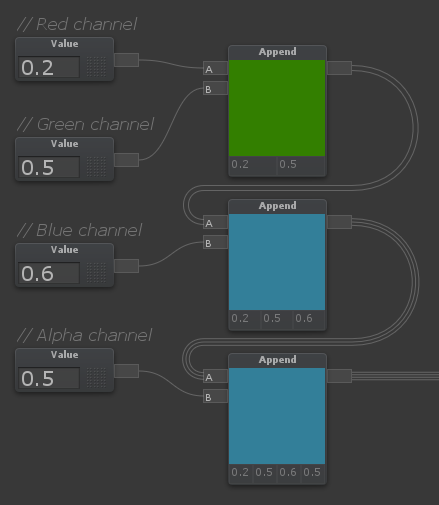Outputs a single vector from multiple input values/vectors. For example, if [A] is a Vector 2, and [B] is a Value (Vector 1), the node will output a Vector 3, where [A] is in the red and green channel, while [B] is in the blue channel
Component Mask
C + LMB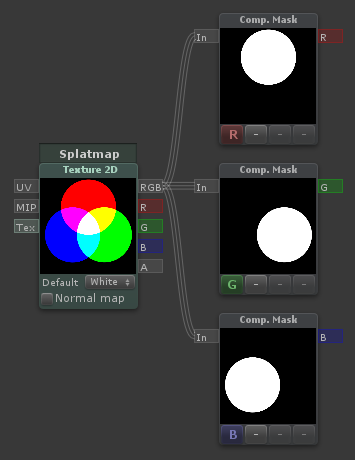The component mask can be used to reorder or extract channels of a vector
DesaturateOutputs a desaturated version of the input [Col]. [Des] Determines how desaturated it is. A value of 1 means fully desaturated, 0.5 means half-desaturated, 0 means no desaturation
Channel Blend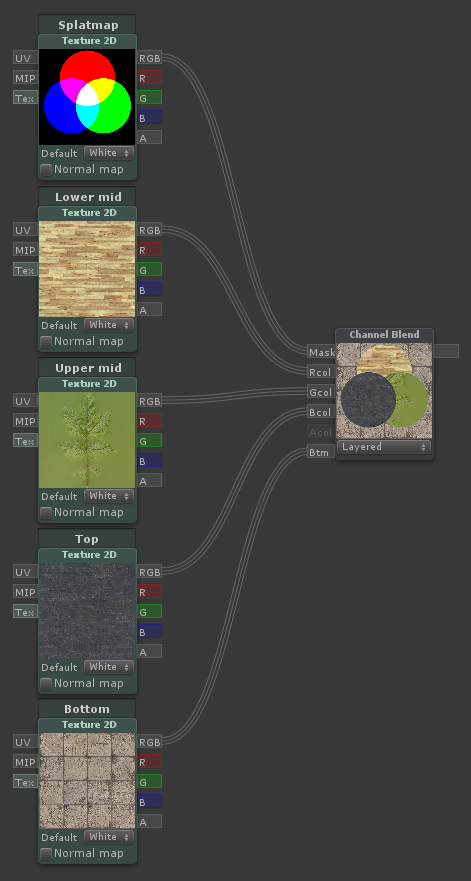Outputs the sum of each component of the mask multiplied by the corresponding color input. Useful for triplanar blending
Normal Blend
 Combines two tangent-space normal directions, where the base normal is perturbed by the detail normal
Distance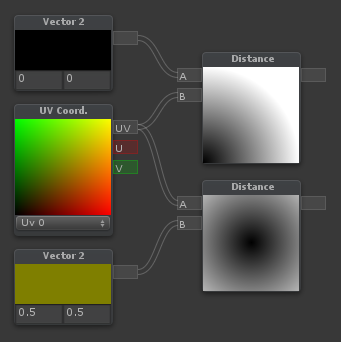Outputs the distance between the two input points [A] and [B]
Length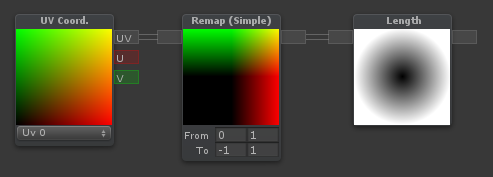Outputs the length/magnitude of its input vector
Transform
 Transforms a vector from one space to another. You can switch between world/local/tangent/view. The built-in vectors are in world space. Directions into the normal input is in tangent space.
Vector Projection
 Outputs vector [A] projected onto vector [B]
Vector Rejection
 Outputs vector [A] rejected from vector [B]
DDX
 Outputs the partial derivative (rate of change) of the input vector in screen space along the X-axis
DDY
 Outputs the partial derivative (rate of change) of the input vector in screen space along the Y-axis
DDXY
 Outputs the absolute sum of the two partial derivatives (rate of change) of the input vector in screen space along the X and Y-axis. Known as fwidth() in code.
Panner
P + LMB
 Outputs the input [UV] coordinates, panned/offset by [Dist] distance, in the direction/speed specified by the U and V parameters
Rotator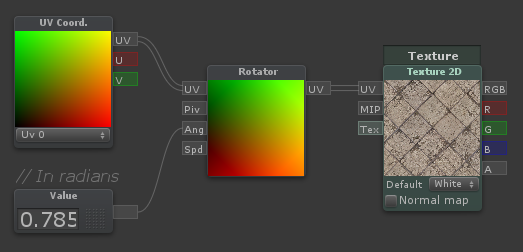Outputs the input [UV] coordinates rotated by [Ang] radians around the pivot point [Piv]. If [Ang] is not connected, or connected to a time node, [Spd] will control the rotation speed
Parallax
 Outputs the input [UV] coordinates with a parallax offset derived from the [Hei] input, with a depth of [Dep] and a reference height [Ref]. A [Ref] height of 0 means it will parallax as if the heightmap sticks out from the mesh A [Ref] height of 1 means it will parallax as if the heightmap goes down into the mesh
UV Tile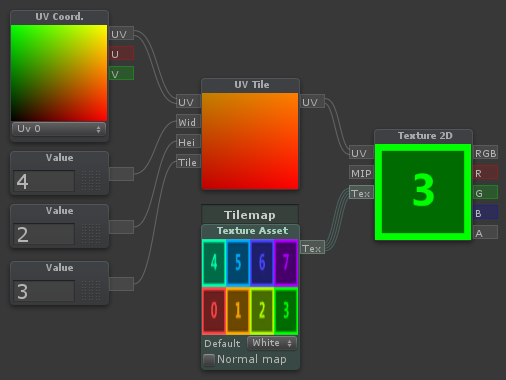UV Tile can be used to get a tile within a tilemap, in the form of UV coordinates. [UV] is the base UVs to get a tile inside [Wid] is the amount of tiles along the tilemap's width [Hei] is the amount of tiles along the tilemap's height [Tile] is the index of the tile in the tilemap you want to extract. Specify this as an integer, where 0 is the first tile, starting from the bottom left.
UV Coordinates
U + LMB
 Outputs the specified UV coordinate for this part of the geometry. The dropdown allows you to select either UV channel 0 or UV channel 1. Note that lightmapped meshes use UV1 for their lightmap UVs
Object Position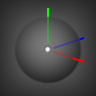Outputs the world position of the object's pivot point
Object Scale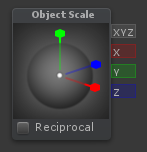Outputs the scale of the object. Checking reciprocal is computationally cheaper, but gives you the inverse scale (1 / scale). Note that this will not work if the mesh is batched.
Screen Position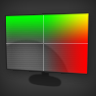Outputs the screen position of the current part of the mesh, can be used as UV coordinates for screen-space mapping. In the dropdown box, "Normalized" will put {0,0} in the center of the screen, and {1,1} in the top right. "Tiled" will keep {0,0} in the center, but will scale on the X axis based on your aspect ratio
World PositionOutputs the position of the current part of the mesh in world space
Vertex Color
V + LMB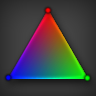Outputs the vertex color. If you've baked ambient occlusion in the vertex colors, or want to tint the mesh with painted colors, or use vertex colors for anything else, this is the node you want
Fresnel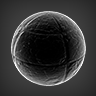Outputs the dot product between the surface normal and the view direction. [Nrm] is used if you want to use a custom normal. By default, the perturbed normal is used. [Exp] changes the exponent of the output. Higher values will make the fresnel thinner
Normal Direction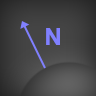Outputs the direction of the mesh normal, in world space. The Perturbed checkbox makes it use the normals after having applied the "Normal" input of the main node, such as a normal map.
Bitangent Direction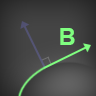Outputs the direction of the mesh bitangent, in world space
Tangent Direction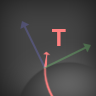Outputs the direction of the mesh tangent, in world space
View Direction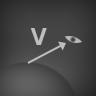Outputs the direction from the current part of the geometry to the camera, in world space
View ReflectionOutputs the direction of the view as if bounced according to the surface normal. This can be used as input for cubemaps for perfect reflections
Face Sign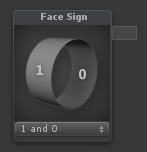This is used to detect if the current face is a frontface or a backface. Outputs 1 on frontfaces, and either -1 or 0 for backfaces, depending on what you specify in the dropdown box.
Light ColorOutputs the color of the current light being rendered
Light AttenuationOutputs the light attenuation. This node contains both the light falloff and shadows in one
Ambient LightOutputs the ambient light of the scene the shader is being rendered in
Light Direction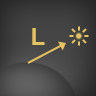Outputs the direction to the current light being rendered
Half Direction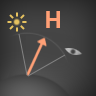Outputs the half-direction. This is the direction that points halfway between the view and the light vector, which is commonly used in the blinn-phong specular model
Light Position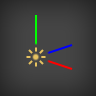Outputs the position of the current light being rendered. [Pnt] Outputs 1 if the current light being rendered is a point light, otherwise it outputs 0
TimeOutputs time at different rates. [t/20] outputs the time running 20 times slower [t] outputs the current time [t*2] outputs the time running twice as fast [t*3] outputs the time running thrice as fast
View Position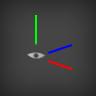Outputs the current location of the view/camera (Note that when using perspective cameras, the view position is "behind" the screen)
Projection Parameters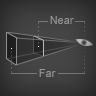Outputs four projection parameters. [Sign] is -1 if currently rendering with a flipped projection matrix, otherwise it's 1 [Near] is the near plane distance of the current camera [Far] is the far plane distance of the current camera [1/Far] is the reciprocal distance of the far plane
Screen Parameters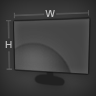Outputs four screen parameters. [pxW] is the width of the screen in pixels [pxH] is the height of the screen in pixels [1+1/W] is 1 plus the reciprocal of the screen pixel width [1+1/H] is 1 plus the reciprocal of the screen pixel height
Pixel Size
 Outputs the size of each pixel as a percentage of the screen width and height. For example, with a resolution of 8x4, this would output a vector with values (0.125, 0.25)
Fog Color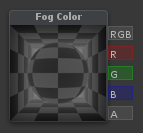Outputs the color of the fog in the current scene
Scene Color
 A texture containing a render of the scene before this object was rendered. By default, its UVs are in screen space, making each pixel represent the color behind the object. This can be used for more advanced blending/transparency effects, or a manual way of making refraction if the UVs are altered, among other things
Scene Depth
 The depth from the camera to the scene behind the current pixel. Note: You have to turn off depth buffer writing in the blend settings, and you need to use a camera that renders a depth texture
Depth Blend
 This outputs a value between 0 and 1, depending on how close this pixel is to the background geometry, based on the distance input. Useful for fading out edges of water, or softening up lightshafts intersecting geometry. Note: You have to turn off depth buffer writing in the blend settings, and you need to use a camera that renders a depth texture
Depth
 This is the distance from the camera's near plane to the object itself
Pi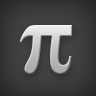Outputs the value of Pi, the ratio of a circle's circumference to its diameter (Approximately 3.141593)
TauOutputs the value of Tau, the ratio of a circle's circumference to its radius (Approximately 6.283185)
Phi (Golden ratio)Outputs the value of Phi, the golden ratio (Approximately 1.618034)
Root 2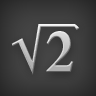Outputs the square root of two (Approximately 1.414214)
e (Euler's Constant)Outputs Euler's constant e (Approximately 2.718282)
Sin
 Outputs the sine of its input
Cos
 Outputs the cosine of its input
Tan
 Outputs the tangent of its input
ArcSin
 Outputs the arcsine of its input, in radians
ArcCos
 Outputs the arccosine of its input, in radians
ArcTan
 Outputs the arctangent of its input, in radians
ArcTan2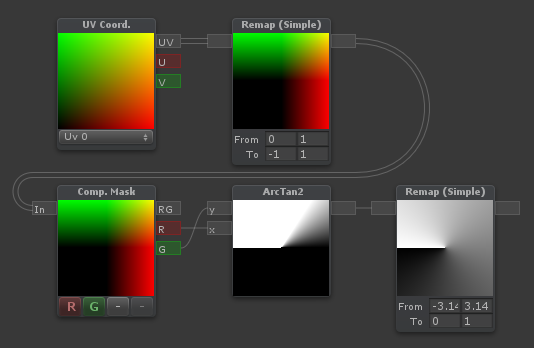Outputs the arctangent of its two inputs coordinates. The range and unit depends on the dropdown box. By default it's in radians, from -pi to pi.
Matrix 4x4A constant matrix. The initial values are that of an identity matrix
Matrix 4x4 PropertyA matrix property. You can assign to it on the C# end using myMaterial.SetMatrix
Multiply Matrix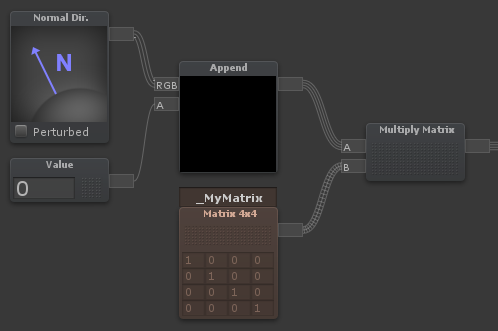A matrix multiply node. Can be used to transform vectors, spaces, or other matrices. You can assign to it on the C# end using myMaterial.SetMatrix(). Note that matrix multiplication is not commutative. That is to say, A * B is not the same thing as B * A
Transpose
 Outputs the transpose of a matrix
CodeA node for doing custom code inside a shader. In the example to the left, the node will work similar to a lerp, that curves towards [mid] before reaching [end]
Relay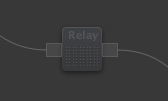Outputs its input, useful for organizing node connections
Set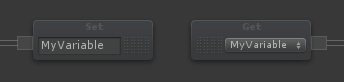Saves a variable to be reused later. Useful for cleaning up large node trees
GetGets one of the previously defined variables using the Set node. Useful for cleaning up large node trees